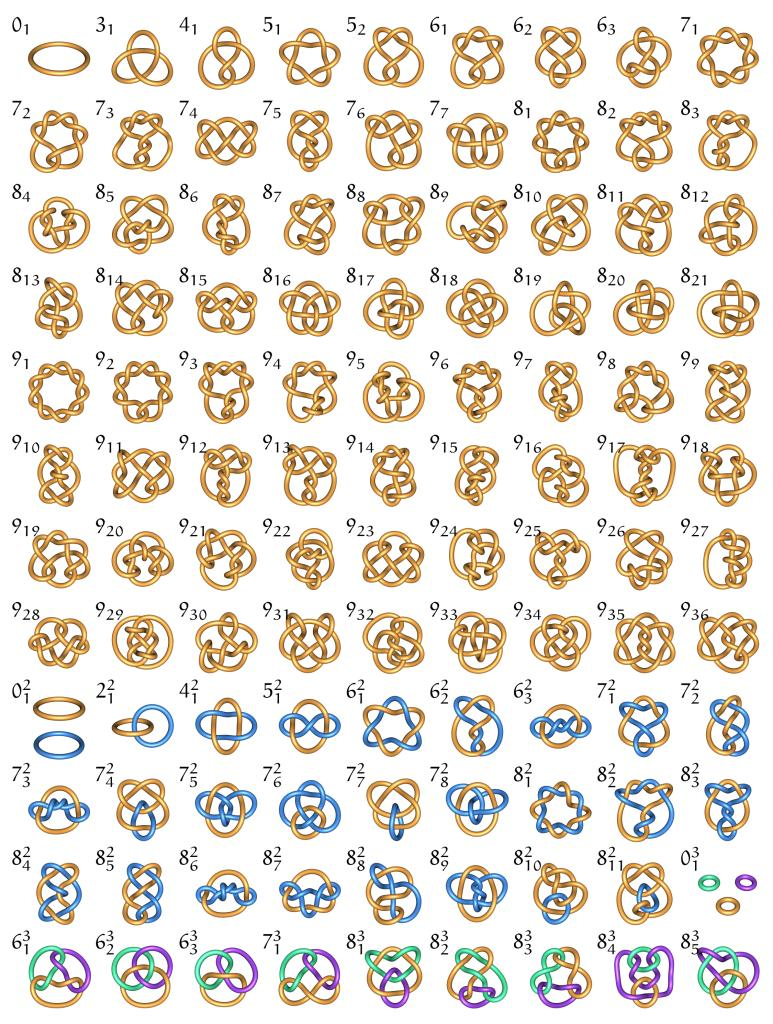## The First Simple Symmetric 11-Venn Diagram

Aug 062012## The Kids Are Alright

Oct 142011

via lewrockwell

Shoutout to Rothbard!

Jul 032011

## Knot Zoo

May 312011via knotzoo

## Math II: Natural Numbers

Dec 272010So, now that we developed an idea of sets, we should start classifying them. We’ll start by constructing the natural numbers.= {0, 1, 2, 3, … }

At this point we only have sets to work with, so we define the empty set as our zero.

0 = ∅ = {}

We take the power set of the empty set and define this set as one.

1 = P(∅) = {∅}

We continue taking power sets to construct the natural numbers

2 = P(P(∅)) = {{∅},∅}

3 = P(P(P(∅))) = {{{∅},∅},{∅},∅}

4 = P(P(P(P(∅))))  …

This gives us a class of sets with a successor operation P which together satisfy the Peano axioms, the essential algebraic properties of the natural numbers. Ie. With this construction mathematicians are able to derive all the known properties of the natural numbers (eg. distributivity).

Even though I’ve ignored some technical details in this construction, this still seems like a lot of work to make the most basic numbers. However, this is an important construction in the foundations of mathematics. Sets are the raw material of math and the natural numbers have significantly more structure. For example, the naturals are well-ordered with unique predecessors. The naturals also admit the binary operations of addition and multiplication. We can’t talk about this structure in a vacuum and that’s why we build these numbers using power sets of the empty set.

We’ll continue from here to talk about the integers and the rational numbers…

Prev: Math I: Sets

Next: Math III: Functions

## Math I: Sets

Dec 262010IMHO mathematics is the study of mathematical elements using the rules of logic. So, the foundational idea of mathematics is a collection of elements known as a set. There are a variety of set theories. For example, naive set theory is perhaps the most intuitive, while ZFC set theory provides the most common axiomatic foundation for math.

The basic idea is that a set is a collection of elements. If a is an element of Z, then we write “a [pmath] in [/pmath] Z”. If the set Z contains the elements a, b, c, then we write this as

Z = {a,b,c}

The number of elements in a set is called the cardinality. The empty set, ∅ = {} , contains no elements and thus has zero cardinality. Next there are finite sets with finite cardinality. Then there are infinite sets of various infinite cardinalities. Georg Cantor hypothesized the continuum hypothesis about possible infinite cardinalities. However, Kurt Godel showed that the continuum hypothesis can neither be proved or disproved using ZFC set theory (Ie. the continuum hypothesis is independent of ZFC).

You can make new sets from existing set using various set operations. For example, if you have one set, you can make subsets or power sets. If you have multiple sets, you can make new sets by using intersections, unions, complements or products. These operations have countless applications. For example, we use power sets to construct the natural numbers and more generally to construct higher cardinalities. We use products of sets to define relations. In particular, the function relation has been used throughout mathematics and the sciences.

From here, I’ll probably write some posts on functions, numbers, infinity, algebra and eventually topology and category theory. I’ll salute Kurt Godel, Georg Cantor and a bunch of other math dudes. Lots of people hated on Cantor for creating set theory and transfinites, but he definitely kept it real throughout.

If you want to solidify your understanding of sets, try these Exercises in Set Theory.

## Wikipedia Warrior

Dec 242010

I am a wikipedia warrior. I love to read the pages. I’m hoping that wikipedia eventually replaces (overpriced) textbooks. I rewrote the alternating series page with gusto. My favorite discoveries since starting this blog are the chimera (genetic) and rational ignorance pages. My favorite quote is naturally from the empty set page:

“Nothing is better than eternal happiness; a ham sandwich is better than nothing; therefore, a ham sandwich is better than eternal happiness.”

As a vegetarian, I guess two slices of white bread is better than eternal happiness. If you’re hungry, please take the swine.

## Empty Set

Nov 122010Hi. Somehow I became a doctor of mathematics. Here’s a copy of my thesis as “proof”. I’m starting this blog as a place to record ideas, interesting papers, etc. The empty set feels like the most natural place to start. 1luv, yall….

 © 2011 ∅ empty set Suffusion theme by Sayontan Sinha